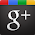### Transpose a matrix using c++ program

This c program prints transpose of a matrix. It is obtained by interchanging rows and columns of a matrix. For example if a matrix is
1 2
3 4
5 6
then transpose of above matrix will be
1 3 5
2 4 6
When we transpose a matrix then the order of matrix changes, but for a square matrix order remains same.

Algorithm :
Matrix Transpose means changing the order or arrangement of a matrix. If A be the given matrix, then the matrix formed by interchanging the columns and rows of the matrix A without changing the value of elements is called as transpose of a matrix. If Am×n = [aij], then AT(n×m) = [aji] i.e. the (i,j)th element of A is the (j,i)th element of AT. So, in A and AT,
• ith column of AT = ith row of A
• jth row of AT = jth column of A
• In A and AT, the diagonal elements are equal.

C++ program code :

Output of program :

Enter the number of Rows of Matrix 1 : 3

Enter the number of Columns of Matrix 1 : 2
Enter the Element a : 1
Enter the Element a : 2
Enter the Element a : 3
Enter the Element a : 4
Enter the Element a : 5
Enter the Element a : 6

Matrix elements :
1 2
3 4
5 6

Matrix elements :
1 3 5
2 4 6

Date - 1/26/2013-@author Y.ACHCHUTHAN -cpp90.blogspot.com
Press any key to continue . . .

1.easy program..

2.basic is easy....

3.Appreciation for nice Updates, I found something new and folks can get useful info about BEST ONLINE TRAINING